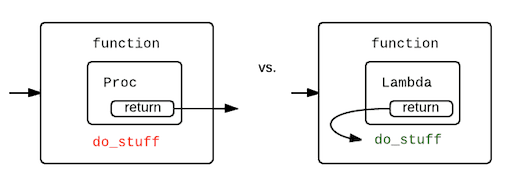Ruby procs vs lambdaIn Ruby, a lambda is a type of anonymous function, similar to a proc. The main difference between a lambda and a proc is that a lambda enforces the number of arguments passed to it, while a proc does not.

Here's an example of how to define a lambda in Ruby:

``` ```
add = lambda { |x, y| x + y }
```
```

And here's an example of how to define a proc:

``` ```
add = proc { |x, y| x + y }
```
```

Both the lambda and the proc can be called in the same way:

``` ```
```
```

There are a few other differences between lambdas and procs in Ruby, such as how they handle the return keyword and how they can be converted to blocks. However, the main difference is the way they handle arguments.

Lambdas will raise an error if you pass the wrong number of arguments to them, while procs will ignore extra arguments and assign nil to any missing arguments. For example:

``` ```
# With a lambda:
add = lambda { |x, y| x + y }

# With a proc:
add = proc { |x, y| x + y }
```
```

In general, it's a good idea to use lambdas when you need to enforce the number of arguments passed to a function, and to use procs when you want more flexibility in the number of arguments.

Our Technology Stack

Work with future-proof technologies